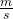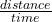## How fast is wave moving if its wavelength is 3m and its frequency is 6 Hz?

Question

How fast is wave moving if its wavelength is 3m and its frequency is 6 Hz?

in progress 0
2 months 2021-08-01T04:09:31+00:00 1 Answers 2 views 0

1. The speed of the wave is 18.

Explanation:

Given : FREQUENCY (F) = 6Hz

WAVELENGTH(λ) = 3m

If a wave has frequency, f, of 6 Hz, its period, T, iss. The wavelength,  λ
, is 3 meters. That means that in the time of one period, the wave travels 3 m.

That means that in the time of one period, the wave travels 3 m.

In General ,

speed =In applying this general definition of speed↑to a wave, we have

speed of the wave=wavelength period

Note: we generally use v for speed of a wave. Using the variable names, then that last formula is written

v=λ ×T

so  speed of the wave = 3× 6 =18